Updating search results...

# 8 Results

View
Selected filters:
• equal shares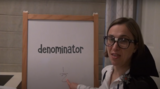Unrestricted Use
CC BY
Rating
0.0 stars

This lesson is Day 10 in a series of 12 lessons around fraction equivalences and comparisons.

This video lesson serves as a review of equpartitioning in order to determine the fractional part of an area or length. This is a necessary foundational skill for comparing and finding equivalent fractions.

Subject:
Mathematics
Material Type:
Activity/Lab
Interactive
Lesson
Simulation
Author:
Dawne Coker
06/25/2020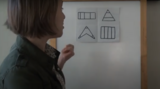Unrestricted Use
Public Domain
Rating
0.0 stars

This lesson is Day 1 in a series of 12 lessons around fraction equivalences and comparisons.

This video lesson encourages students to reason about and visualize fractions. The lesson focuses on the ideas that fractions are fair shares and that fractions can be compared. This lesson assumes that students have some initial experiences with fractions.

Subject:
Mathematics
Material Type:
Interactive
Lesson
Presentation
Simulation
Author:
Dawne Coker
06/25/2020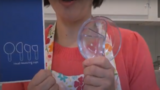Unrestricted Use
CC BY
Rating
0.0 stars

This lesson is Day 4 in a series of 12 lessons around fraction equivalences and comparisons.

This video lesson looks at how we use fractions in the kitchen. Students are challenged to use circle models to find equivalent fractions for a recipe. Students may use virtual circe models from the Toy Theater (https://toytheater.com/fraction-circles/).

Subject:
Mathematics
Material Type:
Activity/Lab
Interactive
Lesson
Simulation
Author:
Dawne Coker
06/25/2020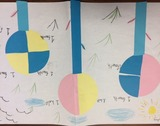Unrestricted Use
CC BY
Rating
0.0 stars

This resource is from Tools4NCTeachers.In this lesson, students partition circles into halves, thirds and fourths as they create fraction flowers.&nbsp; Student recording sheets and sample pictures of student work are included within the lesson.&nbsp; Links to virtual tools and extension ideas are also provided.&nbsp;&nbsp;Remix this lesson to include pictures of your students&#39; work!

Subject:
Mathematics
Material Type:
Activity/Lab
Lesson
Lesson Plan
Author:
DAWNE COKER
06/24/2020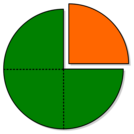Unrestricted Use
CC BY
Rating
0.0 stars

This resource is from Tools4NCTeachers.&nbsp;&nbsp;In this lesson students are introduced to the vocabulary associated with partitioning shapes into equal shares. Students will also explore shapes that are partitioned into halves, thirds, and fourths. Student recording sheets and pictures of anchor charts are included within this lesson.&nbsp;

Subject:
Mathematics
Material Type:
Activity/Lab
Lesson
Lesson Plan
Author:
DAWNE COKER
06/24/2020Unrestricted Use
CC BY
Rating
0.0 stars

This resource is from Tools4NCTeachers.&nbsp;This file contains a set of 4&nbsp;tasks (including scoring rubric,&nbsp;recording sheet, printable materials, and student work samples).&nbsp; The tasks may be&nbsp;used for instruction or assessment.&nbsp;The focus of standard G.3 is on partitioning circles and rectangles into 2, 3, and 4 equal shares.&nbsp; Most of this resource focuses on partitioning rectangles.&nbsp; Please remix this resource to add additional circle tasks. Sharing is caring!

Subject:
Mathematics
Material Type:
Activity/Lab
Assessment
Formative Assessment
Author:
DAWNE COKER
06/16/2020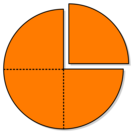Unrestricted Use
CC BY
Rating
0.0 stars

This resource is from Tools4NCTeachers.&nbsp;In this lesson, students explore partitioning shapes into halves and fourths.&nbsp; The lesson could be taught consecutively in one math block or extended over multiple days depending on students&rsquo; understanding of the concept of halves and fourths.

Subject:
Mathematics
Material Type:
Activity/Lab
Lesson
Lesson Plan
Author:
DAWNE COKER
06/12/2020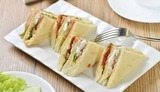Unrestricted Use
CC BY
Rating
0.0 stars

This resource is from Tools4NCTeachers.&nbsp;&nbsp;In this lesson, students will determine ways to partition a sandwich into two equal parts and determine if the halves are the same size.&nbsp; Then students will work with partners to determine different ways to partition a sandwich into fourths and prove that the fourths are equal shares. Sample student work and recording sheets are provided within this lesson.Remix this lesson to share extension activities and related math centers.

Subject:
Mathematics
Material Type:
Activity/Lab
Lesson
Lesson Plan
Author:
DAWNE COKER